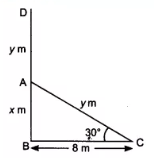Q

# A tree breaks due to storm and the broken part bends so that the top of the tree touches the ground making an angle 30° with it. The distance between the foot of the tree to the point where the top touches the ground is 8 m. Find the height of the tree.

2. A tree breaks due to storm and the broken part bends so that the top of the tree touches the ground making an angle 30° with it. The distance between the foot of the tree to the point where the top touches the ground is 8 m. Find the height of the tree.

ViewsSuppose DB is a tree and the AD is the broken height of the tree which touches the ground at C.
GIven that,
, BC = 8 m
let AB =  m and AD =

In right angle triangle ,

So,  the value of  =

Similarily,

the value of  is

So, the total height of the tree is-

= 8 (1.732) = 13.856 m (approx)

Exams
Articles
Questions